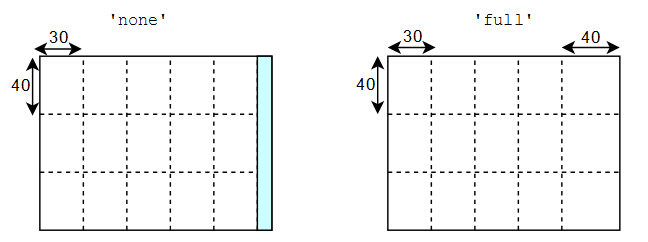# visionhdlframetoregions

Convert video frame dimensions into tiled regions of interest

Since R2020b

## Syntax

``regions = visionhdlframetoregions(activePixelsPerLine,activeLines,numHorTiles,numVerTiles)``
``regions = visionhdlframetoregions(activePixelsPerLine,activeLines,numHorTiles,numVerTiles,'numPix',4)``
``regions = visionhdlframetoregions(activePixelsPerLine,activeLines,numHorTiles,numVerTiles,'fillType','full')``

## Description

example

````regions = visionhdlframetoregions(activePixelsPerLine,activeLines,numHorTiles,numVerTiles)` converts an `activePixelsPerLine`-by-`activeLines` video frame into `numHorTiles`×`numVerTiles` nonoverlapping regions valid for vertical reuse with the ROI Selector block or the `visionhdl.ROISelector` System object™. By default, the function returns a set of equally sized regions and ignores any remainder pixels at the right and bottom of the frame.```
````regions = visionhdlframetoregions(activePixelsPerLine,activeLines,numHorTiles,numVerTiles,'numPix',4)` returns regions that are compatible with multipixel streaming. You can set `numPix` to `4` or `8`.```
````regions = visionhdlframetoregions(activePixelsPerLine,activeLines,numHorTiles,numVerTiles,'fillType','full')` returns regions that cover the entire frame size. If remainder pixels at the right and bottom of the frame exist, the function extends the right and bottom regions to include the remainder pixels.In this diagram, the frame on the left shows the default behavior of the function. The frame is 160-by-120 pixels, divided into five horizontal and three vertical tiles. The function returns a set of fifteen 30-by-40 pixel regions and ignores the 10 remainder pixels at the right of the frame (blue).In this diagram, the frame on the right shows the regions returned when you specify the `'filltype','full'` argument. Similar to the previous case, the frame is 160-by-120 pixels, divided into five horizontal and three vertical tiles. The function returns twelve 30-by-40 pixel regions, and three 40-by-40 regions that include the remainder pixels at the right of the frame.```

## Examples

collapse all

This example shows how to divide a frame into tiled regions of interest (ROIs) and use those regions to configure the ROI Selector block for vertical reuse.

Vertical reuse means dividing each frame into vertically-aligned regions where each column of regions shares a pixel stream. This arrangement enables parallel processing of each column, and the reuse of downstream processing logic for each region in the column.

Set up the size of the frame.

```frmActiveLines = 240; frmActivePixels = 320; ```

Divide the frame into equally-sized vertically-aligned regions, or tiles. The `visionhdlframetoregions` function returns an array of such regions, where each region is defined by four coordinates, and is of the form [ hPos vPos hSize vSize ]. These tile counts divide evenly into the frame dimensions, so no remainder pixels exist. The output regions cover the entire frame.

```numHorTiles = 2; numVerTiles = 2; regions = visionhdlframetoregions(frmActivePixels,frmActiveLines,numHorTiles,numVerTiles) ```
```regions = 1 1 160 120 161 1 160 120 1 121 160 120 161 121 160 120 ```

The ROI Selector block in the Simulink model has the Reuse output ports for vertically aligned regions parameter selected, and uses the `regions` variable to define its output streams. The block has one output pixel stream per column of regions.

```open_system('TiledROIHDL') ```The start and end signals define each region in the pixel stream. When you run the model, you can see the output tiled regions changing in the Left Viewer and Right Viewer windows. The example performs opposite gamma correction operations on the left and right tiles, and then reassembles the four tiles into a complete frame by manipulating the `pixelcontrol` signals.

The blanking interval required by the downstream processing algorithm must be less than the interval between tiles. The blanking interval after each region is less than one line of pixels, so operations that require a vertical blanking interval, like those that use a line buffer, do not work. The gamma correction operation uses a lookup table that does not require a blanking interval.

```sim('TiledROIHDL') ```## Input Arguments

collapse all

Number of pixels in a horizontal line of the active input frame, specified as a positive integer. The function divides this dimension into `numHorTiles` columns. Any remainder pixels from this division are handled according to the `'filltype'` setting.

Data Types: `single` | `double` | `int8` | `int16` | `int32` | `int64` | `uint8` | `uint16` | `uint32` | `uint64` | `fi`

Number of horizontal lines in the active input frame, specified as a positive integer. The function divides this dimension into `numVerTiles` rows. Any remainder pixels from this division are handled according to the `'filltype'` setting.

Data Types: `single` | `double` | `int8` | `int16` | `int32` | `int64` | `uint8` | `uint16` | `uint32` | `uint64` | `fi`

Number of tiles in the horizontal direction, specified as a positive integer. The function returns `numHorTiles`×`numVerTiles` regions. This value must be in the range [1, 16].

Data Types: `single` | `double` | `int8` | `int16` | `int32` | `int64` | `uint8` | `uint16` | `uint32` | `uint64` | `fi`

Number of tiles in the vertical direction, specified as a positive integer. The function returns `numHorTiles`×`numVerTiles` regions. This value must be in the range [1, 1024].

Data Types: `single` | `double` | `int8` | `int16` | `int32` | `int64` | `uint8` | `uint16` | `uint32` | `uint64` | `fi`

## Output Arguments

collapse all

Tiled regions of interest, returned as a `numHorTiles`×`numVerTiles`-by-4 matrix. Each region is represented by four positive integers that define the coordinates of the top-left corner and dimensions of the region, ```[hPos vPos hSize vSize]```. Use these regions to configure the ROI Selector block or `visionhdl.ROISelector` System object for vertical reuse. Regions in each column share an output pixel stream of the block or System object.

## Version History

Introduced in R2020b